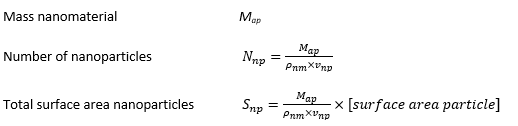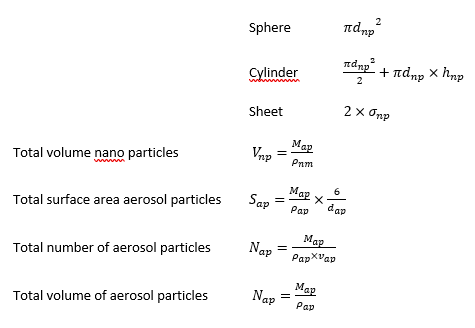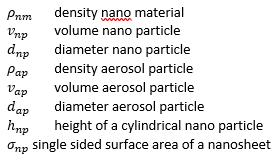# Calculation of the dose metric

The exposure, deposition and clearance models express the output in terms of the mass of the aerosol particles and the model evaluation does not depend on the characteristics of the nanomaterial. Consequently, the different dose metrics can be calculated from the lung load expressed as mass of the deposited aerosol particles Map (g).

The aerosol particle is assumed to consist completely of nanoparticles, which means that no other components are included.

Dose metrics are calculated as:The surface area of the individual particle is calculated from the specification of the nanomaterial geometry by the user. ConsExpo nano supports three particle geometries: sphere, cylinder and sheet. For these, the surface areas are calculated as:These calculations are based on the single valued (i.e. median) aerosol diameter and single valued nano material particle size.

When distributions of aerosol particle and nano particle diameters are considered, calculations change.